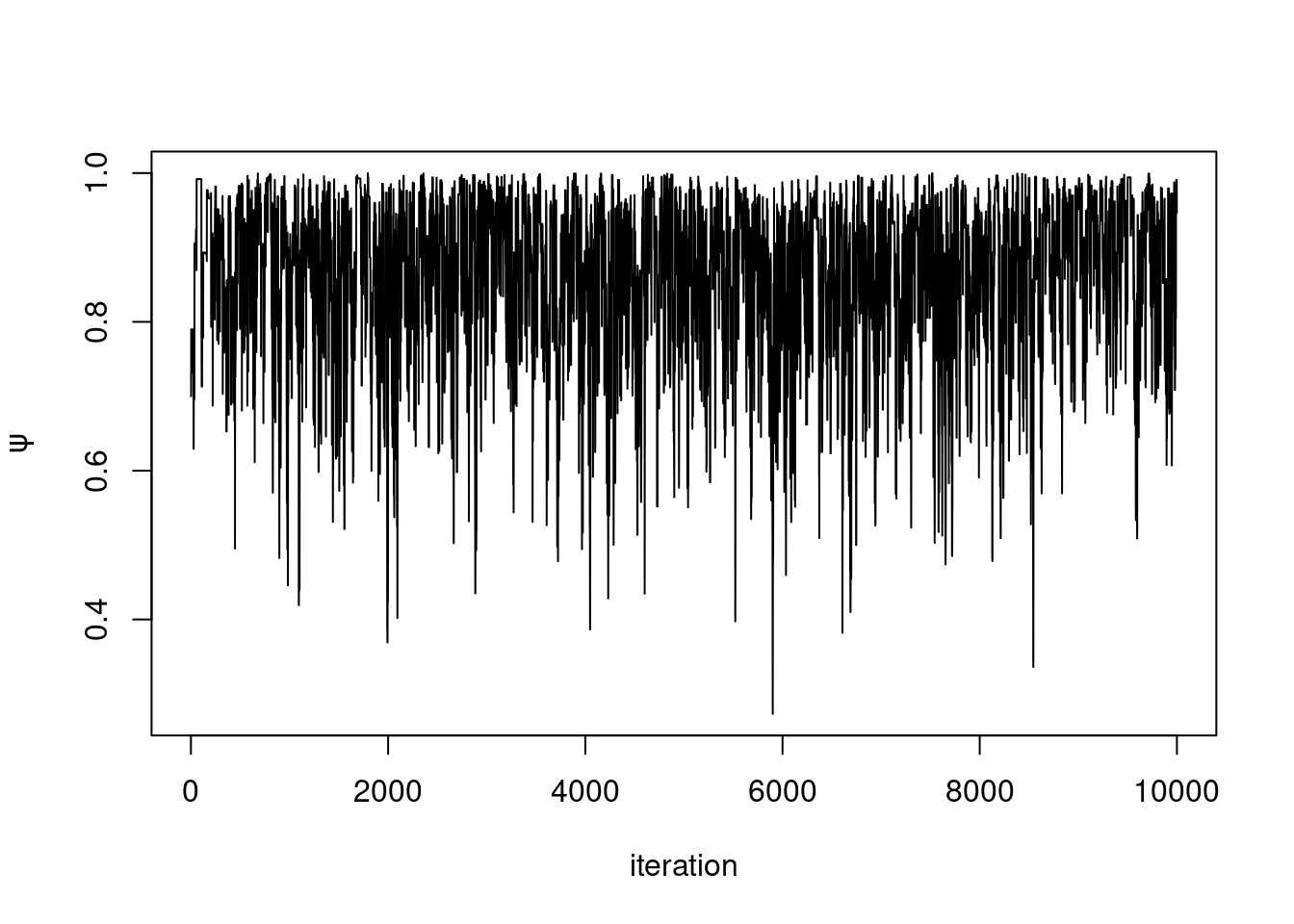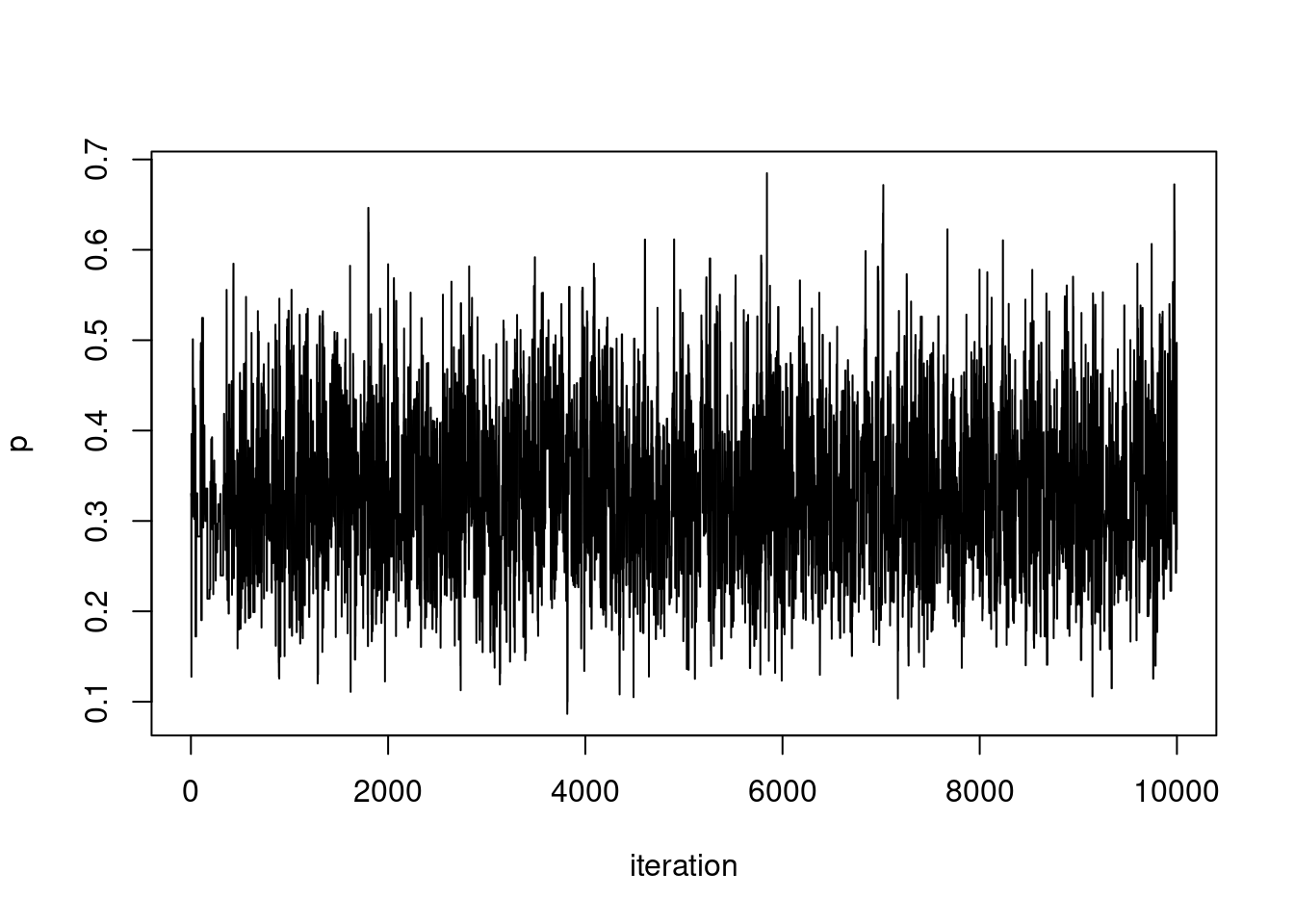# Simulating from a model

NIMBLE models can be used to simulate data. The steps required to do so are as follows:

1. Create BUGS model code with nimbleCode() describing the model
2. Build the model with nimbleModel() providing constants and initial values for parameters needed for simulation
3. Identify the nodes to simulate
4. Run model$simulate() ### 1. Create model code We’ll use a very simple toy hierarchical model, a basic occupancy model from ecology. The occupancy model describes a situation where an observer visits multiple sites multiple times, and on each site visit records whether or not a target species is seen there. The parameters of interest are psi, the probability that any one site is occupied, and p, the probability of detecting the species on any visit given that it is present. Note that the detection probability may depend on the visit but for this simple example we make it a constant across visits. It’s assumed that sites don’t change occupancy status during the visiting period. library(nimble, warn.conflicts = FALSE) ## nimble version 0.12.2 is loaded. ## For more information on NIMBLE and a User Manual, ## please visit https://R-nimble.org. simCode <- nimbleCode({ for (i in 1:nsite) { z[i] ~ dbern(prob = psi) # True occupancy status for (j in 1:nvisit) { y[i,j] ~ dbern(z[i] * p) # Observed data } } psi ~ dunif(0,1) p ~ dunif(0,1) }) ### 2. Build the model We build the model object. We provide constants, in this case, number of sites (nsite) and number of visits to each site (nvisit). We also provide initial values for p and psi. simModel <- nimbleModel(code = simCode, constants = list(nsite = 10, nvisit = 3), inits = list(psi = 0.7, p = 0.33)) ## Defining model ## Building model ## Setting data and initial values ## Running calculate on model ## [Note] Any error reports that follow may simply reflect missing values in model variables. ## Checking model sizes and dimensions ## [Note] This model is not fully initialized. This is not an error. ## To see which variables are not initialized, use model$initializeInfo().
##          For more information on model initialization, see help(modelInitialization).

Note that the message lets us know that some variables are not initialized – in this case, all y and z nodes. If we try to calculate the log probabilities of these nodes at this point, we’ll get NA.

nodesToSim <- simModel$getDependencies(c("psi", "p"), self = F, downstream = T) nodesToSim ##  "z" "z" ##  "z" "z" ##  "z" "z" ##  "z" "z" ##  "z" "z" ##  "lifted_z_oBi_cB_times_p_L4" "lifted_z_oBi_cB_times_p_L4" ##  "lifted_z_oBi_cB_times_p_L4" "lifted_z_oBi_cB_times_p_L4" ##  "lifted_z_oBi_cB_times_p_L4" "lifted_z_oBi_cB_times_p_L4" ##  "lifted_z_oBi_cB_times_p_L4" "lifted_z_oBi_cB_times_p_L4" ##  "lifted_z_oBi_cB_times_p_L4" "lifted_z_oBi_cB_times_p_L4" ##  "y[1, 1]" "y[1, 2]" ##  "y[1, 3]" "y[2, 1]" ##  "y[2, 2]" "y[2, 3]" ##  "y[3, 1]" "y[3, 2]" ##  "y[3, 3]" "y[4, 1]" ##  "y[4, 2]" "y[4, 3]" ##  "y[5, 1]" "y[5, 2]" ##  "y[5, 3]" "y[6, 1]" ##  "y[6, 2]" "y[6, 3]" ##  "y[7, 1]" "y[7, 2]" ##  "y[7, 3]" "y[8, 1]" ##  "y[8, 2]" "y[8, 3]" ##  "y[9, 1]" "y[9, 2]" ##  "y[9, 3]" "y[10, 1]" ##  "y[10, 2]" "y[10, 3]" By setting self = FALSE we specified that we didn’t want to see psi or p in the return vector. By setting downstream = TRUE we recursively looked at the dependencies of nodes, rather than just the nodes directly dependent on psi and p. We successfully retrieved all z and y nodes, as well as an internal set of nodes NIMBLE created to handle the operation z[i] * p. ### 4. Simulate simModel$simulate(nodesToSim)
simModel$y ## [,1] [,2] [,3] ## [1,] 0 0 0 ## [2,] 0 1 0 ## [3,] 0 0 1 ## [4,] 0 1 1 ## [5,] 1 0 0 ## [6,] 0 0 0 ## [7,] 0 0 1 ## [8,] 0 0 0 ## [9,] 0 0 1 ## [10,] 0 1 0 We got values back for y! We can also view the simulated latent state z. simModel$z
##   0 1 1 1 1 1 1 1 1 1

## Compiled models

Note that this workflow looks the same with a compiled model:

CsimModel <- compileNimble(simModel)
## Compiling
##   [Note] This may take a minute.
##   [Note] Use 'showCompilerOutput = TRUE' to see C++ compilation details.
nodesToSim <- CsimModel$getDependencies(c("psi", "p"), self = F, downstream = T) CsimModel$simulate(nodesToSim)

CsimModel$y ## [,1] [,2] [,3] ## [1,] 0 0 1 ## [2,] 0 0 1 ## [3,] 0 1 0 ## [4,] 0 0 0 ## [5,] 0 0 1 ## [6,] 0 0 1 ## [7,] 1 0 0 ## [8,] 0 1 1 ## [9,] 0 0 0 ## [10,] 0 1 0 ## Use simulated data in MCMC To use a simulated dataset for MCMC, the data must be retrieved and set so that the target node is identified as a data node type. Note that we do not set the latent state z, which should not be treated as data by the MCMC algorithm. simulatedYs <- CsimModel$y
CsimModel\$setData(list(y = simulatedYs))

simMCMC <- buildMCMC(CsimModel)
## ===== Monitors =====
## thin = 1: p, psi
## ===== Samplers =====
## RW sampler (2)
##   - psi
##   - p
## binary sampler (10)
##   - z[]  (10 elements)
CsimMCMC <- compileNimble(simMCMC, project = simModel)
## Compiling
##   [Note] This may take a minute.
##   [Note] Use 'showCompilerOutput = TRUE' to see C++ compilation details.
samples <- runMCMC(CsimMCMC, niter = 10000)
## Running chain 1 ...
## |-------------|-------------|-------------|-------------|
## |-------------------------------------------------------|
plot(samples[ , 'psi'], type = 'l', xlab = 'iteration',  ylab = expression(psi))plot(samples[ , 'p'], type = 'l', xlab = 'iteration', ylab = expression(p))